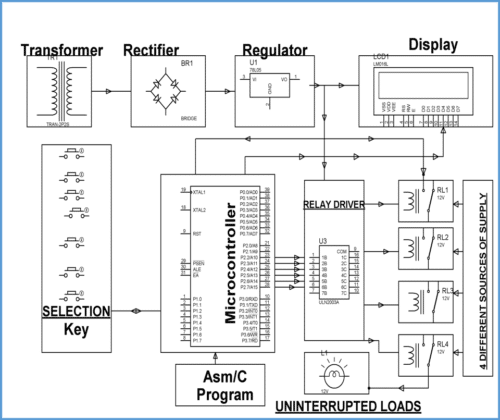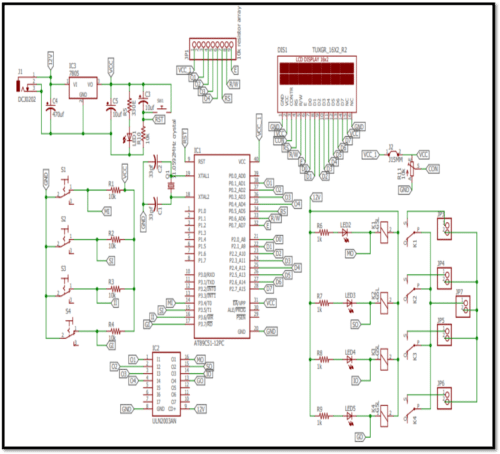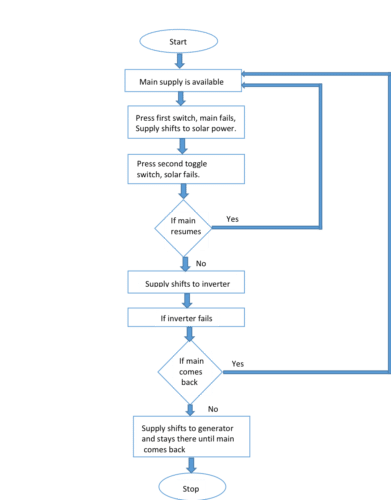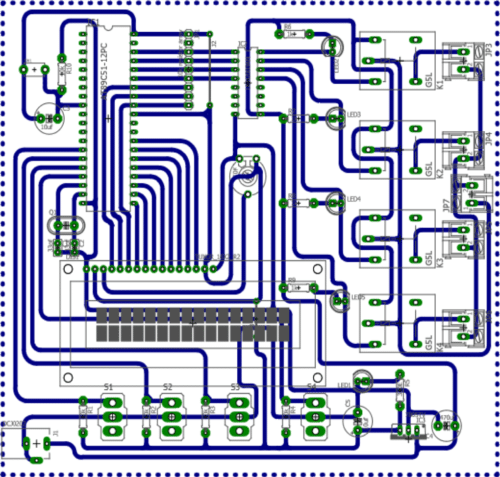# Auto Power Switching Mains, Solar Inverter or Generator

by Aman Sinha, Gaurav Tiwari, Jil Patel, Shivani Shah with Prof. Geetali Saha

15067

13) Interrupt Recovery from Power-down Mode
14) Watchdog Timer
15) Dual Data Pointer
16) Power-oﬀ Flag
17) Fast Programming Time
18) Flexible ISP Programming (Byte and Page Mode)
19) Green (Pb/Halide-free) Packaging Option

## Block Diagram### Working of the Model:

#### The Model works as follows:

This project uses an arrangement of four different sources of supply, which are channelizedto a load to have an uninterrupted operation of the load. As System starts, it shows the Present available Supply on the LCD Screen. Then according to the Priority Predefined in the Program,switching of the Sources takes place. This system works on the pre-arranged priorities as Main Supply, Solar Supply, Inverter Supply, and Generator Supply.Four toggle switches represent four different sources respectively and are interfaced to the controller. The system decision takes place by the commands given to the relay driver i.e. ULN2003 by the programmed Microcontroller AT89S52. Accordingly, the relay driver selects which relay isto be energized.

Initially we have given high input signal to the microcontroller, so as a result the controller generates a low output to activate the first relay driver, which will result in the relay being energized and the LED glows.

Switching takes place as follows:

1) Initially Main Supply is on so all other power sources will be off as Main supply is given the highest priority.
2) As soon as main turns off or power cuts off, Solar Supply will automatically be selected and the system will run on Solar Supply.
3) Now if Main as well as Solar Supply are not available, then inverter is automatically selected and the system runs on Inverter Supply.
4) Finally, if Main Supply, Solar Supply, and Inverter Supply are not available, then the system will automatically switch to Generator and will run on the same.
5) Now amongst all these above-mentioned sources, if any of the source of higher priority comes back, then the system will automatically switch to that particular higher priority source.

LCD interfaced with the module displays the present Source of Energy.

## Schematic Diagram## Flowchart## Software Used

1) Autodesk Eagle
– PCB Designing

2) Keil µvision
-To Program AT89S52## Code Skeleton

#include
#define lcd P2
sbit m=P3^4;
sbit s=P3^5;
sbit in=P3^6;
sbit g=P3^7;
sbit O1=P0^1;
sbit O2=P0^2;
sbit O3=P0^3;
sbit O4=P0^4;
sbit RS=P0^5;
sbit RW=P0^6;
sbit EN=P0^7;
void delay (unsigned intms) // Delay function
{
unsignedinti, j;
for (i=0; i<=ms; i++)
for (j=0; j<500; j++);
}
voidlcd_cmd(unsigned char x) //Lcd command function
{
lcd = x;
RS = 0;
RW = 0;
EN = 1;
delay(10);
EN = 0;
}
voidlcd_data(unsigned char t) // Lcd Data function
{
lcd = t;
RS = 1;
RW = 0;
EN = 1;
delay(10);
EN = 0;
}
voidlcd_initial() //Lcd initialization function
{
lcd_cmd(0x80);
lcd_cmd(0x38); //Function set: 8-bit, 2-line 5×7 dots
lcd_cmd(0x0c); //Display ON, cursor OFF
// lcd_cmd(0x0E); //Display ON, cursor ON

}

voiddisp_str(unsigned char *p) // Display String function
//*p is pointer variable
{
for(*p=0;*p!=’\0′;*p++)
{
lcd_data(*p);
}
}
void main()
{
unsigned char z;
// This initialization is to add different conditions according to your priority//
while(1)
{
if(m==1)
{
O1=1;
O2=0;
O3=0;
O4=0;
z=1;
}
if(m==…..)
{
}
// So according to the condition below mentioned task is going to be excuted
if(z==1)
{
lcd_initial();
//lcd_cmd(0x01); //Clear Display
disp_str(“MAIN POWERSUPPLY”);
lcd_initial();
}
if(z==…..)
{

1.Smitha
•Hiren
2.manmohan singh bisht